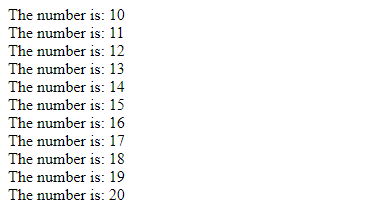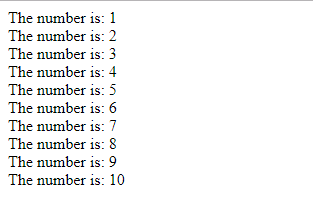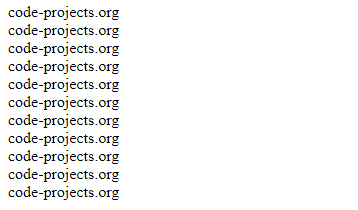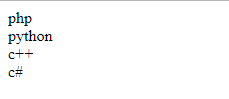# Loops – PHP Basics

## Loops – PHP BasicsLoop is used whenever you want a code to run over and over again in row. We use Loop instead of adding almost same codes many times.

Loop Statements :

Loop continues as long as the condition is true – while
Loop continues a code once and repeats loop until specified condition is true – do..while
Loop continues for a number of time – for
Loop continues block of code for each element in an array – foreach

While Loop :

```<!DOCTYPE html>
<html>
<body>

<?php
\$x = 10;

while(\$x <= 20) {
echo "The number is: \$x <br>";
\$x++;
}
?>

</body>
</html>```

Output :do..while Loop :

```<!DOCTYPE html>
<html>
<body>

<?php
\$x = 1;

do {
echo "The number is: \$x <br>";
\$x++;
} while (\$x <= 10);
?>

</body>
</html>```

Output :for Loop :

```<!DOCTYPE html>
<html>
<body>

<?php
for (\$a = 0; \$a <= 10; \$a++) {
echo "code-projects.org <br>";
}
?>

</body>
</html>```

Output :foreach Loop :

```<!DOCTYPE html>
<html>
<body>

<?php
\$lang = array("php", "python", "c++", "c#");

foreach (\$lang as \$value) {
echo "\$value <br>";
}
?>

</body>
</html>```

Output :